Like   Tweet   Pin   +1   in

## The Healing Power of Art

 /* styles */ Art has anecdotally been found to help people experiencing pain and/or trauma relieve their stress and find hope. Now the research is catching up. Americans for the Arts research has made the case that arts in healthcare intervention reduces hospital stays and decreases the use of sedatives, while improving the general quality of life. The WHO recently completed research compiling evidence from a wide variety of studies on how the arts can potentially impact both mental and physical health.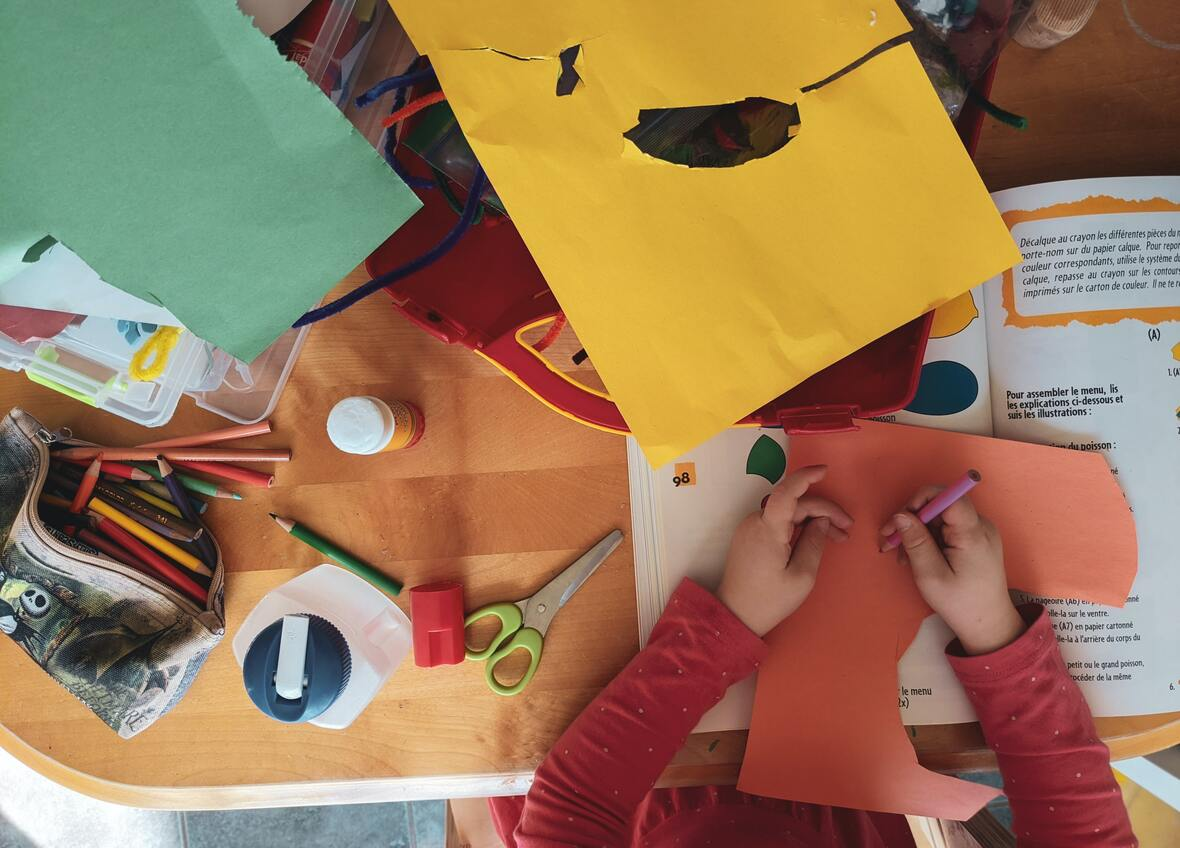/* styles */ The arts in health can range from formal application and training of Art Therapy (our local Cedar Crest College has an Art Therapy program) to the less formal Art in Health (Penn State is partnering with Center Stage to provide asynchronous trainings virtually) initiative where practicing artists can come in support healing through creativity. The Lehigh Valley is fortunate enough to have two top medical intuitions in Lehigh Valley Health Network and the St. Luke's University Health Network, who are continuing to expand their work with the arts. Read on through this issue to learn more about the Arts in Health. Let's work together to bring the arts, artists, caregivers, and patients together to make a healthier region.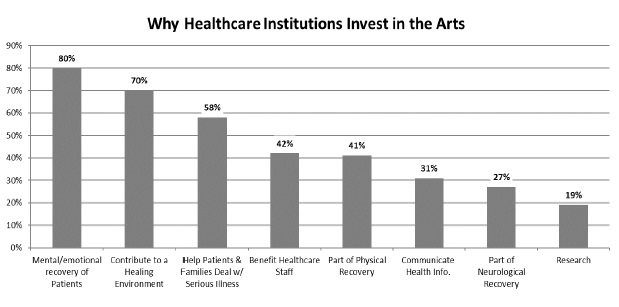/* styles */ Tara Henninger Operations Manager Elise Schaffer Arts & Access Always Program Admin Lehigh Valley Arts Council
 table div table+table+table+table+table+table+table+table div table{width:100%;padding:0}table div table+table+table+table+table+table+table+table div table img{width:96.23%;padding:0;float:none}table div table+table+table+table+table+table+table+table div table td{width:100%;padding:0 1.88% 18px}/* styles */# Online Arts Calendar

Enjoy arts experiences online and in-person in the Lehigh Valley. View our online arts calendar and see what's scheduled for this Spring and Summer!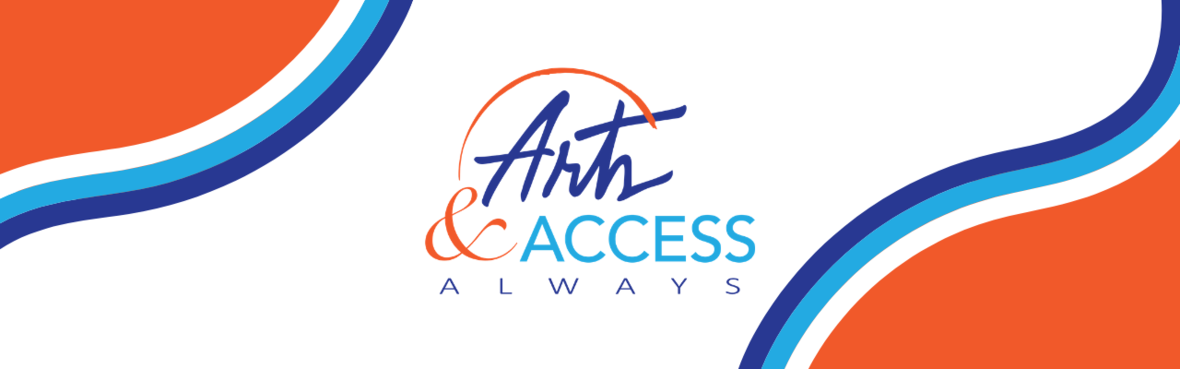# Arts & Access Always | Google Classroom

 /* styles */ Are you interested in accessibility resources? Our program is also excited to share our brand new Google Classroom where you can find resources, connect with local arts administrators, and share information on arts and accessibility. You will need a Google account to check it out.
 table div table+table+table+table+table+table+table+table+table+table+table+table+table+table+table div table{width:100%;padding:0}table div table+table+table+table+table+table+table+table+table+table+table+table+table+table+table div table img{width:96.23%;padding:0;float:none}table div table+table+table+table+table+table+table+table+table+table+table+table+table+table+table div table td{width:100%;padding:0 1.88% 18px}/* styles */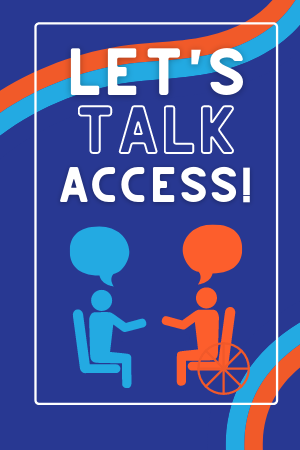# Arts Access Networking

Our Arts & Access Always Program is so excited to offer our first-ever networking group! Meet monthly with local arts administrators, artists, and students to discuss accessibility initiatives in their organizations, share resources, and information, and connect with local organizations that serve the disability community. Our next meeting will be held in July! You can get the Zoom link and more information about the group by emailing our Arts & Access Always Program Coordinator, Elise, by clicking the button below.

 table div table+table+table+table+table+table+table+table+table+table+table+table+table+table+table+table+table+table div table{width:100%;padding:0}table div table+table+table+table+table+table+table+table+table+table+table+table+table+table+table+table+table+table div table img{width:96.23%;padding:0;float:none}table div table+table+table+table+table+table+table+table+table+table+table+table+table+table+table+table+table+table div table td{width:100%;padding:0 1.88% 18px}/* styles */# Disability-Friendly Calendar

 table.module-19{width:99.81%;padding:0}table div table+table+table+table+table+table+table+table+table+table+table+table+table+table+table+table+table+table+table+table div table{width:99.81%;float:none;margin-left:auto;margin-right:auto;padding:0}table div table+table+table+table+table+table+table+table+table+table+table+table+table+table+table+table+table+table+table+table div table a{border:0 none;text-decoration:none}table div table+table+table+table+table+table+table+table+table+table+table+table+table+table+table+table+table+table+table+table div table img{width:100%!important;border:0 none;text-decoration:none}table div table+table+table+table+table+table+table+table+table+table+table+table+table+table+table+table+table+table+table+table div table td{width:100%;padding:0}/* styles */
 /* styles */ Are you looking for accessible events this summer? Check out our disability-friendly calendar for new listings including some audio-described Shakespeare Festival performances and a special event with Disability Dialogue at ArtsWalk in Allentown! Visit the calendar on our website.# Local Opportunities

## Hope & Healing Juried Art Show

 table div table+table+table+table+table+table+table+table+table+table+table+table+table+table+table+table+table+table+table+table+table+table+table+table div table,table.module-23{width:3.77%;float:right;padding:0}table div table+table+table+table+table+table+table+table+table+table+table+table+table+table+table+table+table+table+table+table+table+table+table+table div table a{border:0 none;text-decoration:none}table div table+table+table+table+table+table+table+table+table+table+table+table+table+table+table+table+table+table+table+table+table+table+table+table div table img{width:100%!important;border:0 none;text-decoration:none}table div table+table+table+table+table+table+table+table+table+table+table+table+table+table+table+table+table+table+table+table+table+table+table+table div table td{width:100%;padding:0 0 20px 20px}/* styles */ NOW ACCEPTING ART for 2022 show: Before December 1, 2021: Register to participate. They will communicate directly with registered teachers and students regarding important updates.
 table div table+table+table+table+table+table+table+table+table+table+table+table+table+table+table+table+table+table+table+table+table+table+table+table+table div table{width:100%;padding:0}table div table+table+table+table+table+table+table+table+table+table+table+table+table+table+table+table+table+table+table+table+table+table+table+table+table div table img{width:96.23%;padding:0;float:none}table div table+table+table+table+table+table+table+table+table+table+table+table+table+table+table+table+table+table+table+table+table+table+table+table+table div table td{width:100%;padding:0 1.88% 18px}/* styles */# State & National Opportunities

## National Endowment for the Arts Grants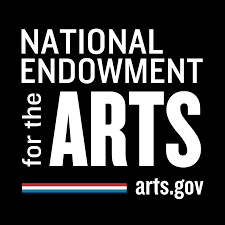The National Endowment for the Arts (NEA) is announcing two programs to distribute American Rescue Plan (ARP) funds. These programs are open to nonprofit arts and culture organizations and local arts agencies, regardless of whether they have received NEA funding in the past.

 table div table+table+table+table+table+table+table+table+table+table+table+table+table+table+table+table+table+table+table+table+table+table+table+table+table+table+table+table div table{width:100%;padding:0}table div table+table+table+table+table+table+table+table+table+table+table+table+table+table+table+table+table+table+table+table+table+table+table+table+table+table+table+table div table img{width:96.23%;padding:0;float:none}table div table+table+table+table+table+table+table+table+table+table+table+table+table+table+table+table+table+table+table+table+table+table+table+table+table+table+table+table div table td{width:100%;padding:0 1.88% 18px}/* styles */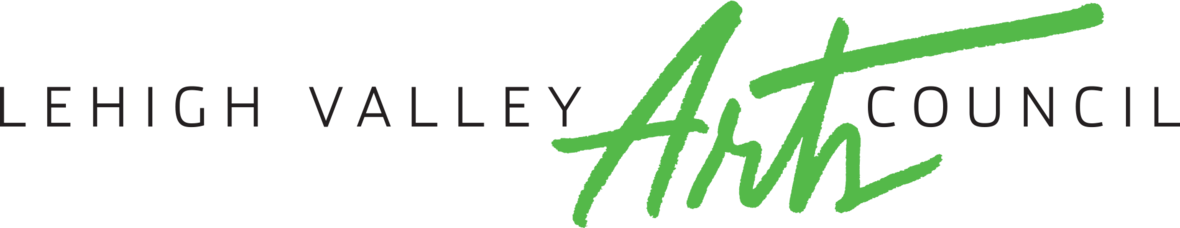## Inside Track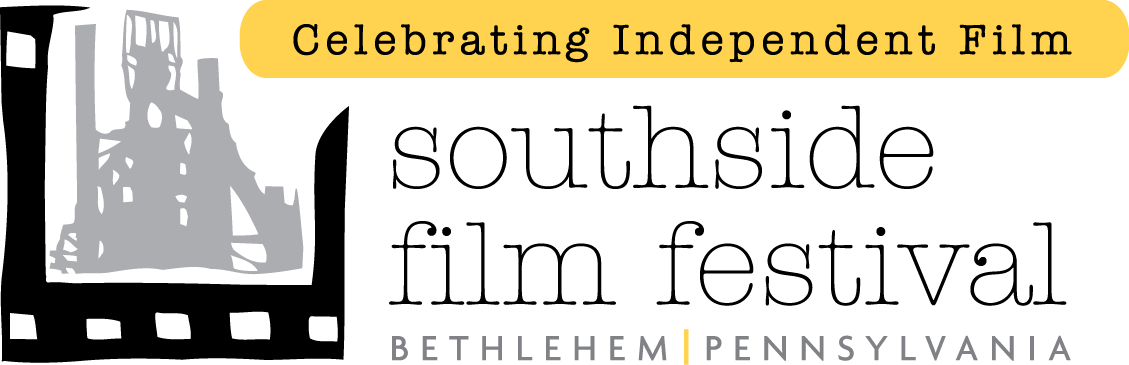/* styles */ Congratulations to the Southside Film Institute on the 17th annual SouthSide Film Festival! The festival was held June 15-19, 2021, and featured over 50 films from around the country and the world! SouthSide Film Institute was a recipient of PPA PROGRAM STREAM 2019 funding./* styles */ Help Citizens for the Arts in Pennsylvania advocate for Pennsylvania to significantly increase support for the ailing arts and culture sector. Please join us in contacting your legislators to urge the passing of a \$10.59 million line item for grants to the arts during FY 2020-21.
 table div table+table+table+table+table+table+table+table+table+table+table+table+table+table+table+table+table+table+table+table+table+table+table+table+table+table+table+table+table+table+table+table+table+table+table+table+table div table{width:100%;padding:0}table div table+table+table+table+table+table+table+table+table+table+table+table+table+table+table+table+table+table+table+table+table+table+table+table+table+table+table+table+table+table+table+table+table+table+table+table+table div table img{width:96.23%;padding:0;float:none}table div table+table+table+table+table+table+table+table+table+table+table+table+table+table+table+table+table+table+table+table+table+table+table+table+table+table+table+table+table+table+table+table+table+table+table+table+table div table td{width:100%;padding:0 1.88% 18px}/* styles */## Research Opportunity

A faculty member at Florida State University is seeking visual and performing artists to participate in a survey that investigates how visual and performing artists construct a meaningful portfolio (freelance) career. The survey is estimated to take approximately 5 to 10 minutes and is anonymous. The voice of visual and performing artists is crucial to this research and I hope you will consider participating! The survey can be accessed by clicking here.# We Couldn't Do This Without You

Please consider making a tax-deductible contribution to our nonprofit arts organization. Together, we can open the door wider for the arts. View our latest donors report on our website.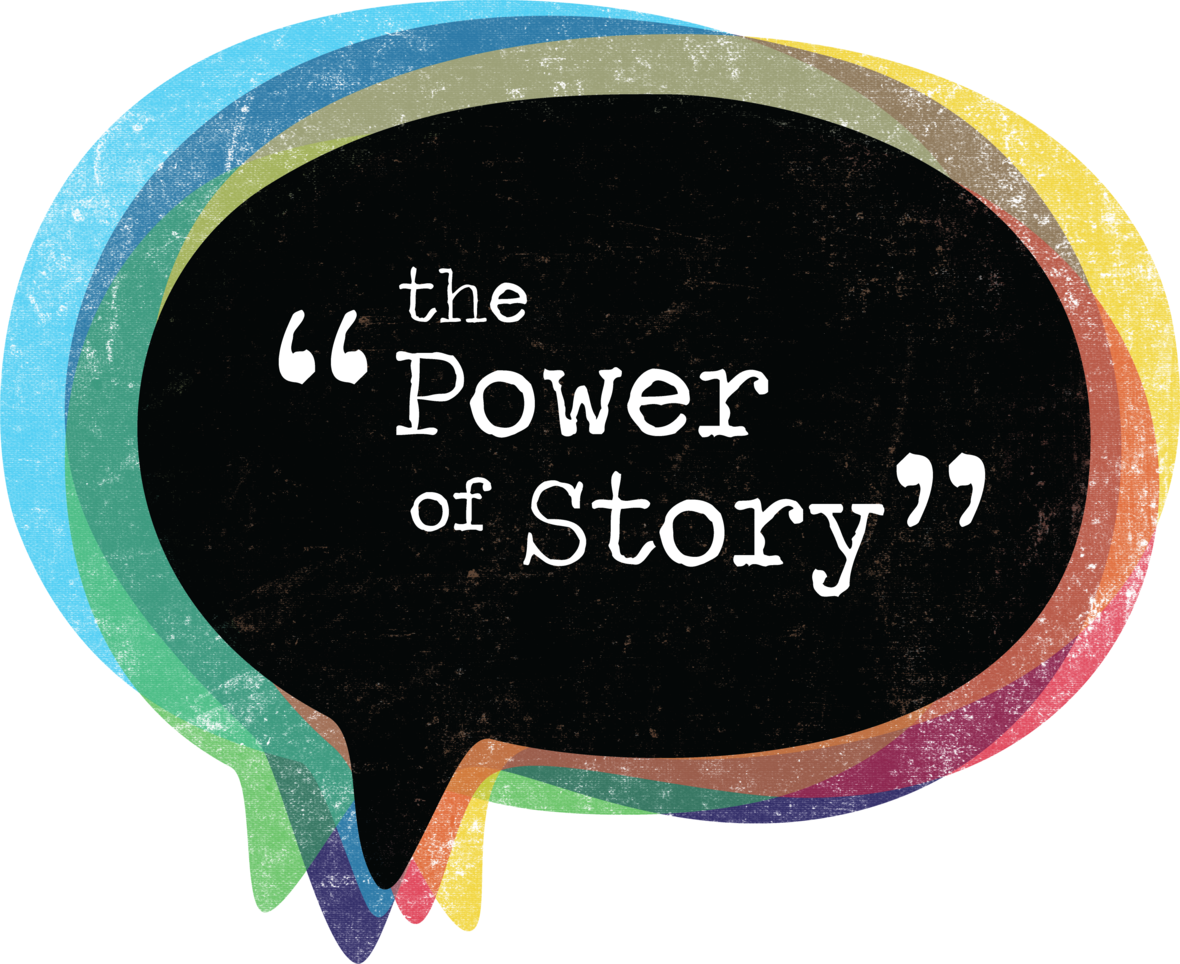# Bonnie Winfield, Art Therapist

A story from inside the walls of the Northampton County Jail during a Journey Home program

## Painting the Pain

One day the pain of being a mother inside these bare walls was unbearable. In our group, we shared our stories. Dr. Bonnie suggested we write the pain in a story as a form of healing for our next group meeting. The next week we witnessed the stories of each member of the group as she read her words. We wept and held hands as we shared the sorrow and pain. After we read our stories, Dr. Bonnie brought out a large piece of watercolor paper, baby wipes, and jars of acrylic paint. She instructed us to express our pain in the colors on the page. With the baby wipes, we painted the colors of our sorrow and joys. As we placed our colors on the page the pain began to be healed. We were amazed at the wonderful colors on the page. The next week, Dr. Bonnie brought the big colorful page back with other art supplies. We finished the piece with words and images. We stood back and admired our creation and knew this was a process of healing.

 table.module-43{width:0%;padding:0}table div table+table+table+table+table+table+table+table+table+table+table+table+table+table+table+table+table+table+table+table+table+table+table+table+table+table+table+table+table+table+table+table+table+table+table+table+table+table+table+table+table+table+table+table div table{width:0%;float:none;margin-left:auto;margin-right:auto;padding:0}table div table+table+table+table+table+table+table+table+table+table+table+table+table+table+table+table+table+table+table+table+table+table+table+table+table+table+table+table+table+table+table+table+table+table+table+table+table+table+table+table+table+table+table+table div table a{border:0 none;text-decoration:none}table div table+table+table+table+table+table+table+table+table+table+table+table+table+table+table+table+table+table+table+table+table+table+table+table+table+table+table+table+table+table+table+table+table+table+table+table+table+table+table+table+table+table+table+table div table img{width:100%!important;border:0 none;text-decoration:none}table div table+table+table+table+table+table+table+table+table+table+table+table+table+table+table+table+table+table+table+table+table+table+table+table+table+table+table+table+table+table+table+table+table+table+table+table+table+table+table+table+table+table+table+table div table td{width:100%;padding:0}/* styles */
 /* styles */ The arts have the power to connect us with one another and sharing stories can help us understand the human experience. Discover personal reflections on the arts in our library, The Power of Story..## National Org for Arts in Health

National Organization for Arts in Health, NOAH provides transformational leadership to bring the field of arts in health together, and to move the field forward. Their focus is on the future of arts, health, and wellbeing; and creating tangible impact from our goals and initiatives.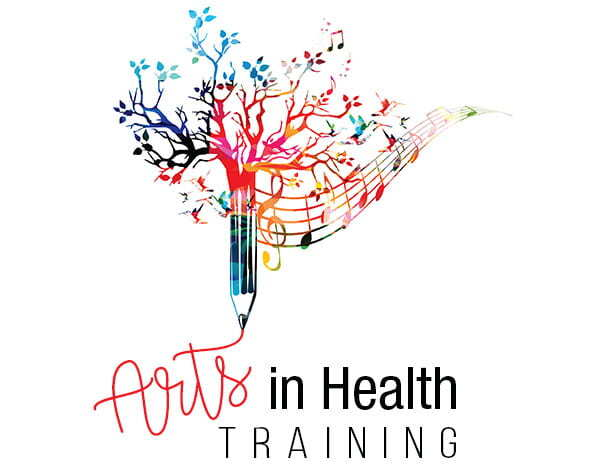## Arts in Health Training

As the field of arts in health grows and professionalizes across the country, Center Stage offers training to welcome and prepare PA artists to work in hospitals. Thanks to a grant from the Pennsylvania Council on the Arts, we have developed an online, asynchronous, free course through the Penn State Health System.## NEA Creative Forces Initiative

Creative Forces: NEA Military Healing Arts Network is an initiative of the National Endowment for the Arts in partnership with the U.S. Departments of Defense and Veterans Affairs and the state and local arts agencies that seeks to improve the health, wellness, and quality of life for military and veteran populations exposed to trauma, as well as their families and caregivers. Read more about this amazing program on their website.

## St. Luke's Healing Arts

St. Luke's Artist-in-Residence works with oncology patients in one-on-one sessions, providing a nurturing and inspirational creative experience.Learn more about St. Luke's Healing Arts program!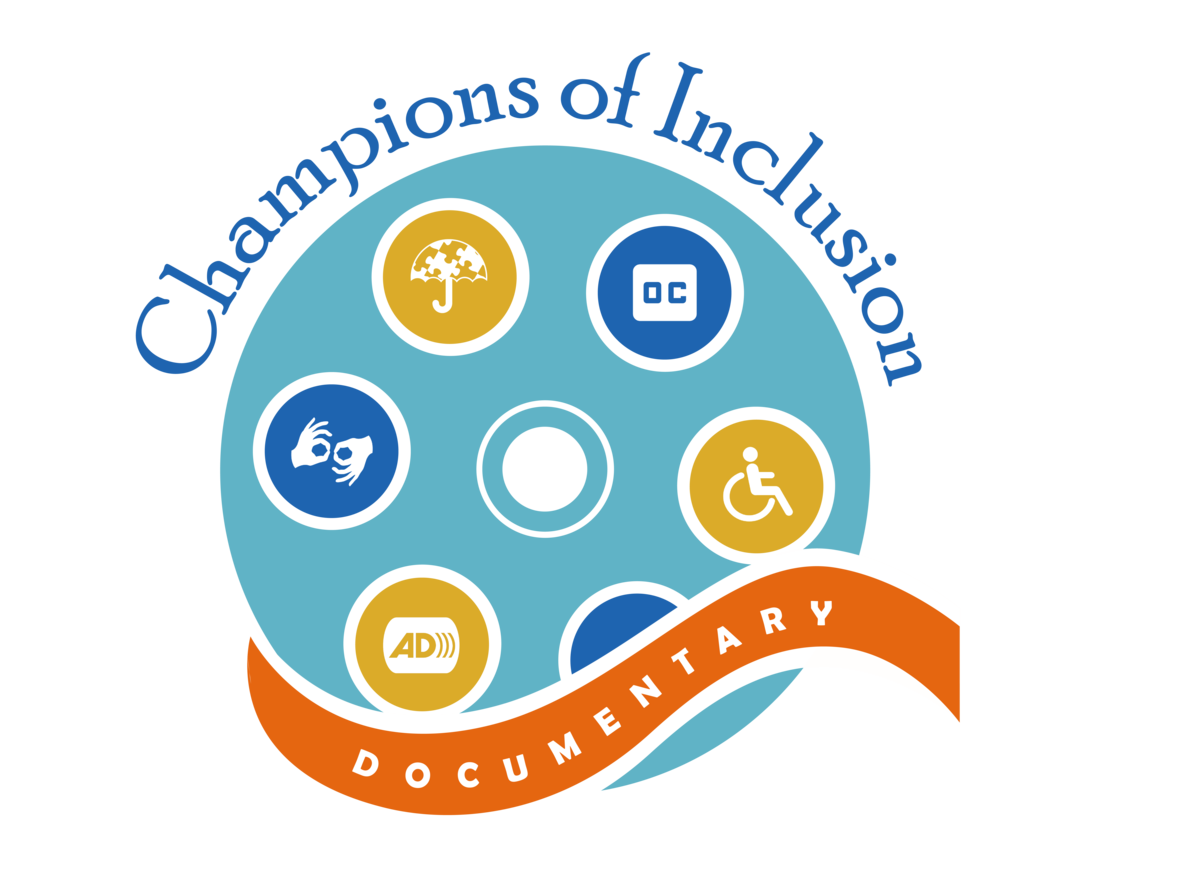## Champions of Inclusion Doc

COVID-19 changed a lot in 2020, so we needed to reimagine our plans. To keep the same energy of connection and learning from our Champions of Inclusion trade fair in 2018, we leaned on the relationships that have developed through our Arts & Access Always program. In this documentary, you’ll meet the mothers and fathers, brothers and sisters, the activists, and the advocates who have nurtured a culture of belonging in the Lehigh Valley. Watch the full documentary online.# Americans with Disabilities Act

## 31st Anniversary of the Passage of the ADA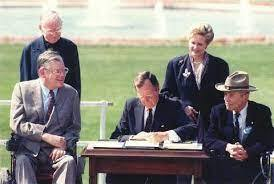table div table+table+table+table+table+table+table+table+table+table+table+table+table+table+table+table+table+table+table+table+table+table+table+table+table+table+table+table+table+table+table+table+table+table+table+table+table+table+table+table+table+table+table+table+table+table+table+table+table+table+table+table+table+table+table+table div table{width:100%;padding:0}table div table+table+table+table+table+table+table+table+table+table+table+table+table+table+table+table+table+table+table+table+table+table+table+table+table+table+table+table+table+table+table+table+table+table+table+table+table+table+table+table+table+table+table+table+table+table+table+table+table+table+table+table+table+table+table+table div table img{width:96.23%;padding:0;float:none}table div table+table+table+table+table+table+table+table+table+table+table+table+table+table+table+table+table+table+table+table+table+table+table+table+table+table+table+table+table+table+table+table+table+table+table+table+table+table+table+table+table+table+table+table+table+table+table+table+table+table+table+table+table+table+table+table div table td{width:100%;padding:0 1.88% 18px}/* styles *//* styles */ Access is a civil right. As the ADA marks its 31th anniversary on July 26, 2021, persons with disabilities also celebrate coming of age politically. They are empowered by the experiences they have undergone. With the passage of this legislation 31 years ago, access became a civil right, and persons with disabilities were no longer isolated or invisible. To learn more about the ADA and its history, click here.
 table div table+table+table+table+table+table+table+table+table+table+table+table+table+table+table+table+table+table+table+table+table+table+table+table+table+table+table+table+table+table+table+table+table+table+table+table+table+table+table+table+table+table+table+table+table+table+table+table+table+table+table+table+table+table+table+table+table+table div table{width:100%;padding:0}table div table+table+table+table+table+table+table+table+table+table+table+table+table+table+table+table+table+table+table+table+table+table+table+table+table+table+table+table+table+table+table+table+table+table+table+table+table+table+table+table+table+table+table+table+table+table+table+table+table+table+table+table+table+table+table+table+table+table div table img{width:96.23%;padding:0;float:none}table div table+table+table+table+table+table+table+table+table+table+table+table+table+table+table+table+table+table+table+table+table+table+table+table+table+table+table+table+table+table+table+table+table+table+table+table+table+table+table+table+table+table+table+table+table+table+table+table+table+table+table+table+table+table+table+table+table+table div table td{width:100%;padding:0 1.88% 18px}/* styles */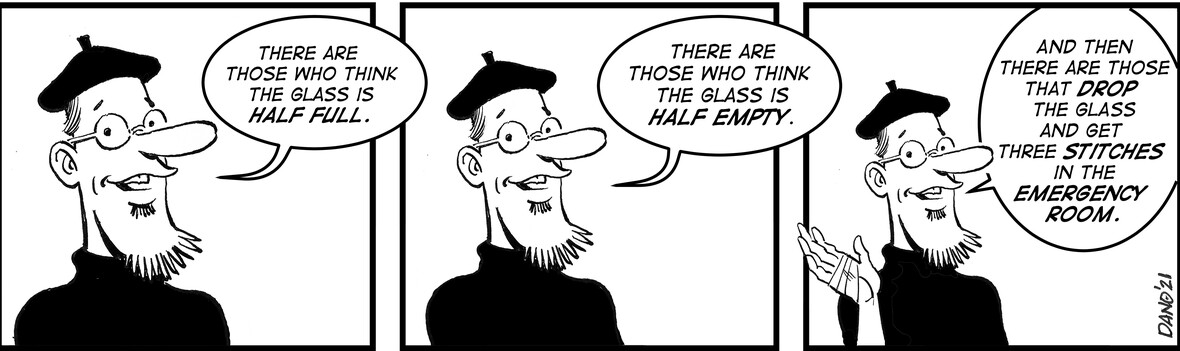/* styles */ Inside the Arts is the new digital version of our traditionally printed bi-monthly newsletter. Topics cover initiatives by the Lehigh Valley Arts Council that impact the regional cultural community.
 /* styles */ Stay in the loop! Conversations on the Arts cross many topics. Subscribe to what you're interested in.
 table.module-62{width:43.02%;padding:0}table div table+table+table+table+table+table+table+table+table+table+table+table+table+table+table+table+table+table+table+table+table+table+table+table+table+table+table+table+table+table+table+table+table+table+table+table+table+table+table+table+table+table+table+table+table+table+table+table+table+table+table+table+table+table+table+table+table+table+table+table+table+table+table div table{width:43.02%;float:none;margin-left:auto;margin-right:auto;padding:0}table div table+table+table+table+table+table+table+table+table+table+table+table+table+table+table+table+table+table+table+table+table+table+table+table+table+table+table+table+table+table+table+table+table+table+table+table+table+table+table+table+table+table+table+table+table+table+table+table+table+table+table+table+table+table+table+table+table+table+table+table+table+table+table div table a{border:0 none;text-decoration:none}table div table+table+table+table+table+table+table+table+table+table+table+table+table+table+table+table+table+table+table+table+table+table+table+table+table+table+table+table+table+table+table+table+table+table+table+table+table+table+table+table+table+table+table+table+table+table+table+table+table+table+table+table+table+table+table+table+table+table+table+table+table+table+table div table img{width:100%!important;border:0 none;text-decoration:none}table div table+table+table+table+table+table+table+table+table+table+table+table+table+table+table+table+table+table+table+table+table+table+table+table+table+table+table+table+table+table+table+table+table+table+table+table+table+table+table+table+table+table+table+table+table+table+table+table+table+table+table+table+table+table+table+table+table+table+table+table+table+table+table div table td{width:100%;padding:0}/* styles */